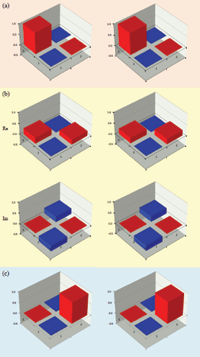## Observation of Fast Evolution in Parity-Time-Symmetric System

Chao Zheng, Liang Hao, Gui Lu LongTo find and realize the optimal evolution between two states is significant both in theory and application. In quantum mechanics, the minimal evolution is bounded by the gap between the largest and smallest eigenvalue of the Hamiltonian. In the parity-time-symmetric(PT-symmetric) Hamiltonian theory, it was predicted that the optimized evolution time can be reduced drastically comparing to the bound in the Hermitian case, and can become even zero. In this Letter, we report the experimental observation of the fast evolution of a PT-symmetric Hamiltonian in an nuclear magnetic resonance (NMR) quantum system. The experimental results demonstrate that the PT-symmetric Hamiltonian can indeed evolve much faster than that in a quantum system, and time it takes can be arbitrary close to zero.

http://arxiv.org/abs/1105.6157
Quantum Physics (quant-ph)